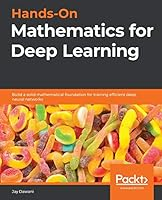# Hands-On Mathematics for Deep Learning: Build a solid mathematical foundation for training efficient deep neural networks0 Reviews
2020-06-12
364 pages

## Book Description

A comprehensive guide to getting well-versed with the mathematical techniques for building modern deep learning architectures

#### Key Features

• Understand linear algebra, calculus, gradient algorithms, and other concepts essential for deep neural networks
• Learn the mathematical concepts needed to understand how deep learning function
• Use deep learning for solving problems related to vision, image, text, and sequence applications

#### Book Description

Most programmers and data scientists struggle with mathematics, having either overlooked or forgotten core mathematical concepts. This book uses Python libraries to help you understand the math required to build deep learning (DL) models.

You'll begin by learning about core mathematical and modern computational techniques used to and implement DL algorithms. This book will cover essential topics, such as linear algebra, eigenvalues and eigenvectors, the singular value decomposition concept, and gradient algorithms, to help you understand how to train deep neural networks. Later chapters focus on important neural networks, such as the linear neural network and multilayer perceptrons, with a primary focus on helping you learn how each model works. As you advance, you will delve into the math used for regularization, multi-layered DL, forward propagation, optimization, and backpropagation techniques to understand what it takes to build full-fledged DL models. Finally, you'll explore CNN, recurrent neural network (RNN), and GAN models and their application.

By the end of this book, you'll have built a strong foundation in neural networks and DL mathematical concepts, which will help you to confidently research and build custom models in DL.

#### What you will learn

• Understand the key mathematical concepts for building neural network models
• Discover core multivariable calculus concepts
• Improve the performance of deep learning models using optimization techniques
• Understand computational and their importance in DL
• Explore the backpropagation to reduce output error
• Cover DL algorithms such as convolutional neural networks (CNNs), sequence models, and generative adversarial networks (GANs)

#### Who this book is for

This book is for data scientists, machine learning developers, aspiring deep learning developers, or anyone who wants to understand the foundation of deep learning by learning the math behind it. Working knowledge of the Python and machine learning basics is required.

1. Linear Algebra
2. Vector Calculus
3. Probability and Statistics
4. Optimization
5. Graph Theory
6. Linear Neural Networks
7. Feedforward Neural Networks
8. Regularization
9. Convolutional Neural Networks
10. Recurrent Neural Networks
11. Attention Mechanisms
12. Generative Models
13. Transfer and Meta Learning
14. Geometric Deep Learning

## Book Details

• Title: Hands-On Mathematics for Deep Learning: Build a solid mathematical foundation for training efficient deep neural networks
• Author:
• Length: 364 pages
• Edition: 1
• Language: English
• Publisher:
• Publication Date: 2020-06-12
• ISBN-10: 1838647295
• ISBN-13: 9781838647292